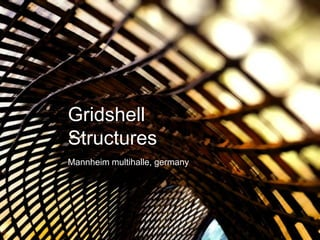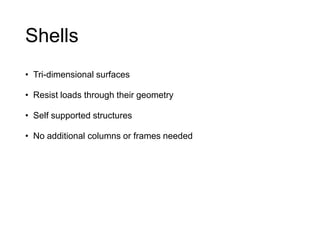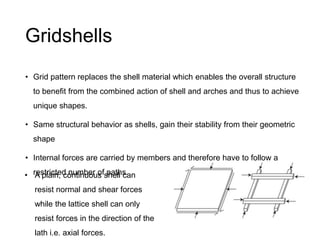Seu SlideShare está sendo baixado. ×

# Understanding Gridshell Structures - Mannheim Multihalle Case Study

Anúncio
Anúncio
Anúncio
Anúncio
Anúncio
Anúncio
Anúncio
Anúncio
Anúncio
Anúncio
AnúncioCarregando em…3
×

1 de 30 Anúncio

# Understanding Gridshell Structures - Mannheim Multihalle Case Study

The Mannheim Multihalle is a physical proof that little more than simple math and a detailed model could be used to create a structure with both organic materials and form.

The Mannheim Multihalle is a physical proof that little more than simple math and a detailed model could be used to create a structure with both organic materials and form.

Anúncio
Anúncio

### Understanding Gridshell Structures - Mannheim Multihalle Case Study

1. 1. • Tri-dimensional surfaces • Resist loads through their geometry • Self supported structures • No additional columns or frames needed
2. 2. • Grid pattern replaces the shell material which enables the overall structure to benefit from the combined action of shell and arches and thus to achieve unique shapes. • Same structural behavior as shells, gain their stability from their geometric shape • Internal forces are carried by members and therefore have to follow a restricted number of paths. • A plain, continuous shell can resist normal and shear forces while the lattice shell can only resist forces in the direction of the lath i.e. axial forces.
3. 3. • The grid was initially flat and the structure was later raised into its doubly-curved shape. • The forces transformed the square grids into similar parallelograms causing the diagonal lines through the nodes to change.
4. 4. • Aesthetics required the cross-section of the laths be 50mm x 50mm. • Increased lath size also increased the initial bending stress. • Engineers decided to double the laths one above the other creating four - rather than two - layers of wooden laths.
5. 5. • Four layers of laths complicated the construction scheme • Members needed to rotate and slide between two parallel laths during the erection process, creating the parallelogram cells. • For this, a pinned connection was needed between the middle two laths and slotted holes were needed in the outer layers to allow for this movement
6. 6. • During its erection, the grid is pushed up from below using scaffolding towers, which induce bending stresses in the laths. • After the mesh is lifted into shape, the boundaries must be fixed. • The pins were tightened with node bolts to retain the shape.
7. 7. • Addition of blocking pieces, known as shear blocks, between parallel laths increased the shell's overall stiffness. • Although the out of plane shear stiffness was provided by bolting and shear blocks, steel cable ties were needed to provide the diagonal stiffness to the shell. • Steel cables of 6mm diameter were tied and stressed by inserting a small block of steel between two parallel cables
8. 8. Hanging chain model • When a uniform distributed load is applied to a suspended line, it naturally shapes itself so as to be free of bending moments. • Chains remain fully in tension • Once inverted, all the internal forces act in compression. • Minimized shear forces.
9. 9. • The Mannheim Multihalle spans 85 meters and contains 7400 m2 of roof area, but its shell thickness is less than half a meter. • The ratio of the thickness of the shell to the span is approximately .00625 which means that the structure is proportionately thinner than an eggshell! • The completed roof structure weighs only 16 kg/m, much lesser than an average concrete plain shell.
10. 10. • The out of plane shear stiffness was provided by bolting and shear blocks while steel cable ties provided the diagonal stiffness to the shell. • The forces flow down to the boundary of the mesh, where the structure has a concrete boundary. • The laths are connected with bolts to a wooden board that is set at the correct angle and connected to concrete blocks using steel brackets. • The boundaries at locations where there are openings were either arches or laminated timber beams which provide the necessary resistance to the lath force without increasing edge thickness, which are often connected to columns.
11. 11. • A simplified structural analysis was performed. • Two forces need to be considered: • The initial force (Pinitial) required to begin deflecting a lath, • The subsequent force (P) required to keep the lath deflected once it is in place, to be referred to as the "lift force." • These two forces were determined (without considering the lath's self-weight) using Timoshenko's elastic stability theory P= Force needed to maintain bent shape of lath
12. 12. • Since the elastic stability theory deals with a cantilevered beam, only half the arc length of the Multihalle was considered. Therefore, l is defined in Equation 1 as: (1) • where L = 80m, and l = 40m. Given this value of l and angle of deflection α of 60 degrees for the Multihalle (based on the arc length and the Multihalle's estimated height of 20 m), the elastic stability theory allows for an estimation of the elliptic integral B that can be used to solve for the lift force P.
13. 13. • For the dimensions of the Multihalle, B = 1.686. Using B, k can be solved by substituting Equation 3 into Equation 2: (2) (3) which, when combined, yield Equation 4 to solve for the lift force P: (4) • The initial force Pinitial can be solved using Equation 5: (5)
14. 14. • Specificity of the construction scheme requires members to be flexible enough so that they can deform during the construction phase. • Materials with a large Young's Modulus, such as steel (E = 210 GPa), are stiffer and require a greater Pinitial and P because more force is needed to bend them. • Materials with a smaller Young's Modulus, such as wood (E~10 GPa) and aluminum (E = 69 GPa), are more flexible and require a smaller Pinitial and P because they bend more easily. • The capacity of timber to bend without braking and to remain elastic makes timber a material of choice.
15. 15. • It is important to note the significant increase in initial force, Pinitial, that occurs if the shear blocks are introduced before erection, due to increased moment of inertia. • For wood, for example, the initial force increases from 0.04 kN to 0.92 kN if the shear blocks are placed prior to erection, which is a significant difference. • These values confirm the ingenuity of the erection process used to construct the Mannheim Pavilion, since the process reduced the overall lift force required without sacrificing the shell's final stiffness.
16. 16. • Once these forces were determined, the stresses experienced by the shell were calculated under four different load cases: • The lift force required to erect the shell lattice • The self-weight of the lattice • The design snow load • The design wind load • For the first and second load cases: (6) where Ia is the moment of inertia of a single lath, since these load cases are resisted by individual laths before they become connected via shear blocks, and ya is equal to 0.25m (perpendicular distance from the edge of a lath to its neutral axis).
17. 17. • The equation used to calculate the stress of the laths in the third and fourth load cases is the following: (7) where Ib is the moment of inertia of two connected laths, joined by a shear block, since these load cases are resisted by the shell as a whole once the shear blocks are in place, and yb is equal to 0.75m (perpendicular distance from the edge of a lath to the neutral axis of the combined laths and shear block).The area is twice as large in this instance because the stress is shared by two laths that are now connected.
18. 18. • Considering that the compressive strength of hemlock is 46.7 MPa, the maximum stresses created by the design loads (~25 MPa) is less than what this type of wood can resist. • The cost of the multipurpose hall was relatively low compared to other typical building projects at the time. • The Mannheim Pavilion is impressive not only for its unique construction process, but also for its design durability despite the technological limits of the time in which it was envisaged and realized. • The Mannheim Multihalle is a physical proof that little more than simple math and a detailed model could be used to create a structure with both organic materials and form.
19. 19. • The design of grid shells structures both in the form finding process and in the prediction behavior of the building under operation appears to be a complex process. • Contrary to other more common structural systems no design guide lines exist and no tools have been developed specially for their study until now and the engineers have resorted to techniques such as chain form finding test their designs. • The fact is, that the lack of expertise in this field is a hindrance to the further development of grid shells.